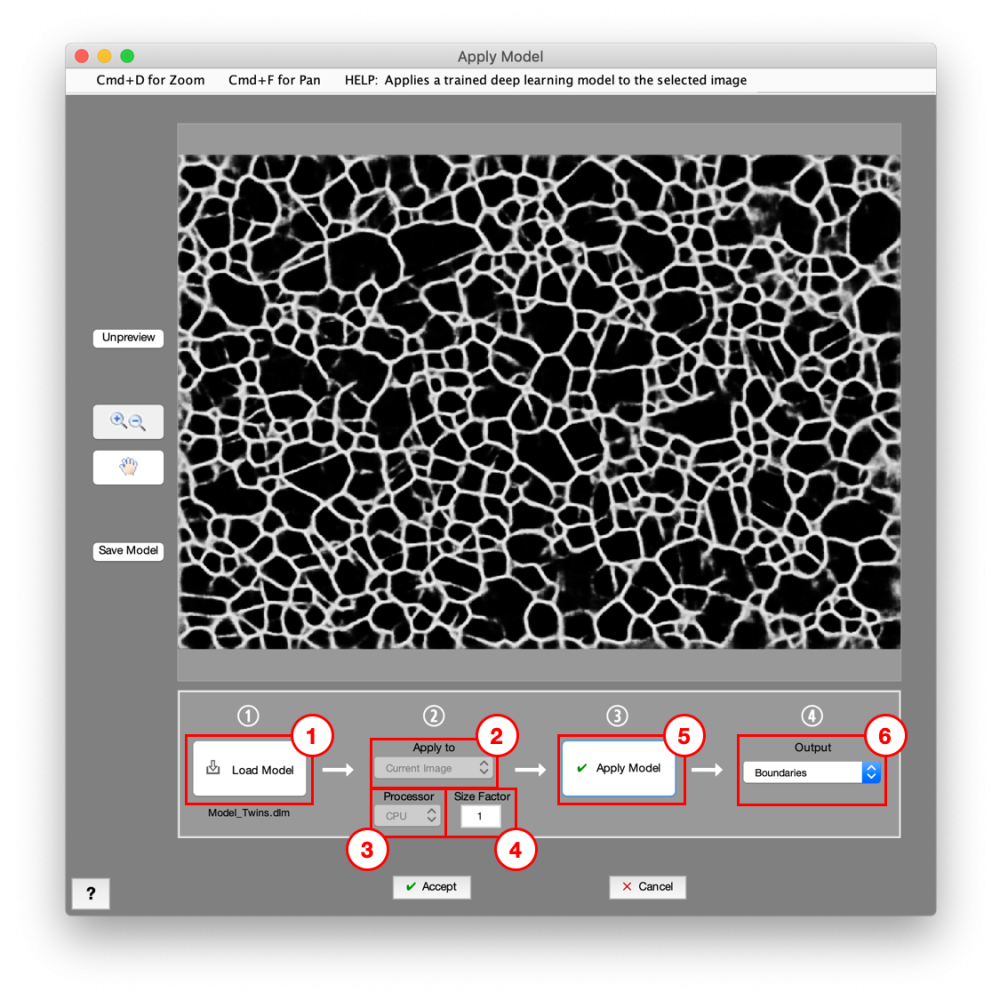### Deep Learning > Apply Model

Applies a trained deep learning model to the selected image. Output can be a probability map of any of the trained layers, or a layer map. A probability map indicates each pixel’s likelihood to belong to that layer. A layer map represents the most likely layer for each pixel.Load deep learning model. These are created and saved from the Deep Learning Trainer.

### 2. Apply To

Choose image to apply model to.

• Color Image: Model is applied to the originally opened color image (only available for color images).
• Current Image: Model is applied to Current Image.

### 3. Processor

Processor to apply model with.

• CPU: Apply model on the CPU.

### 4. Size Factor

Resize factor prior to applying model (0-1). Image will be resized according to the this factor, model applied, then results upsampled back to original size. It is recommended to use the same size factor as was used to train the model.

### 5. Apply Model

Apply deep learning model.

### 6. Output

Choose image to display and output.

• Layer Map: Represents the most likely Layer classification for each pixel.
• Other Options: Probability maps of the trained Layers. A probability map indicates each pixel’s likelihood to belong to that layer.

## Example

Applying a deep learning model

Need more help with this?
Chat with an expert now ››